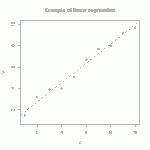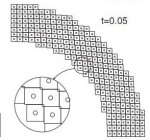# Tutorial: multi-linear regressionThe straight line is the linear regression of a function that takes scalars (x-values) as input and returns scalars (y-values) as output. (figure from GANFYD)

You’ve probably seen classical equations for linear regression, which is a procedure that finds the straight line that best fits a set of discrete points$\{(x_1,y_1), (x_2,y_2),...,(x_N,y_N)\}$. You might also be aware that similar formulas exist to find a straight line that is a best (least squares) fit to a continuous function$y(x)$.The pink parallelogram is the multi-linear regression of a function that takes vectors (gray dots) as input and returns vectors (blue dots) as output

The bottom of this post provides a link to a tutorial on how to generalize the concept of linear regression to fit a function$\vec{y}(\vec{x})$ that takes a vector$\vec{x}$ as input and produces a vector$\vec{y}$ as output. In mechanics, the most common example of this type of function is a mapping function that describes material deformation: the input vector is the initial location of a point on a body, and the output vector is the deformed location of the same point. The image shows a collection of input vectors (initial positions, as grey dots) and a collection of output vectors (deformed locations as blue dots). The affine fit to these descrete data is the pink parallelogram. Continue reading

# Publications: Nonuniqueness and instability of classical formulations of nonassociative plasticityA plot of the frequency-dependent wave propagation velocity for the case study problem with an overlocal plasticity model, with the elastic and local hardening wave speeds shown for reference (left). Stress histories using an overlocal plasticity model with a nonlocal length scale of 1m and a mesh resolution of 0.125m (right)

The following series of three articles (with common authors J. Burghardt and R. Brannon of the University of Utah) describes a state of insufficient experimental validation of conventional formulations of nonassociative plasticity (AKA nonassociated and non-normality).  This work provides a confirmation that such models theoretically admit negative net work in closed strain cycles, but this simple prediction has never been validated or disproved in the laboratory!

# Publication: A convected particle domain interpolation technique to extend applicability of the material point method for problems involving massive deformationsThree snapshots of the model with 248 particles in simulation of the radial expansion of a ring problem using: (a) CPDI method and (b) cpGIMP

A new algorithm is developed to improve the accuracy and efﬁciency of the material point method for problems involving extremely large tensile deformations and rotations. In the proposed procedure, particle domains are convected with the material motion more accurately than in the generalized interpolation material point method. This feature is crucial to eliminate instability in extension, which is a common shortcoming of most particle methods. Also, a novel alternative set of grid basis functions is proposed for efﬁciently calculating nodal force and consistent mass integrals on the grid. Speciﬁcally, by taking advantage of initially parallelogram-shaped particle domains, and treating the deformation gradient as constant over the particle domain, the convected particle domain is a reshaped parallelogram in the deformed conﬁguration. Accordingly, an alternative grid basis function over the particle domain is constructed by a standard 4-node ﬁnite element interpolation on the parallelogram. Effectiveness of the proposed modiﬁcations is demonstrated using several large deformation solid mechanics problems.

Available Online:

http://onlinelibrary.wiley.com/doi/10.1002/nme.3110/abstract

# Verification Research: MMS vortex ring simulation

Below are shown comparisons of the exact and numerical solution for the vortex ring problem on a square domain.Continue reading

# Verification Research: The method of manufactured solutions (MMS)MMS stands for “Method of Manufactured Solutions,” which is a rather sleazy sounding name for what is actually a respected and rigorous method of verifying that a finite element (or other) code is correctly solving the governing equations.

A simple introduction to MMS may be found on page 11 of The ASME guide for verification and validation in solid mechanics. The basic idea is to analytically determine forcing functions that would lead to a specific, presumably nontrivial, solution (of your choice) for the dependent variable of a differential equation.  Then you would verify a numerical solver for that differential equation by running it using your analytically determined forcing function.  The difference between the code’s prediction and your selected manufactured solution provides a quantitative measure of error.